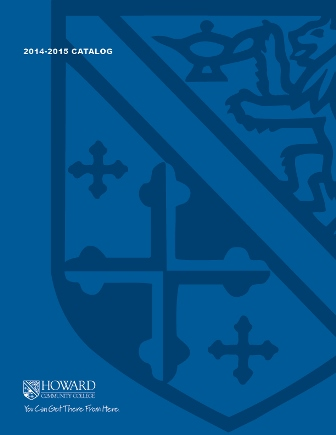2017-2018 College Catalog# MATH 138 Statistics

In this course, students will develop the skills necessary to examine basic statistical terminology, display, describe, and analyze categorical and quantitative data, and calculate measures of central location and variation. The student will additionally examine the normal distribution, correlation, and regression analysis, sampling, hypotheses testing, the chi square test, and probability related to statistics. Classes will require the use of a statistical package.

4

### Prerequisite

MATH 037 or MATH 070 or higher and ENGL 086 or ENGL 096 or higher or appropriate placement scores

4 hours weekly

### Course Objectives

1. 1. Communicate mathematical and statistical concepts using appropriate symbols, notation,
and vocabulary.
2. 2. Analyze categorical and quantitative data using appropriate statistical methods including use
of descriptive and inferential statistics.
3. 3. Evaluate, justify, and interpret observations and inferences derived from statistical methods.
4. 4. Assess the validity of statistical arguments and conclusions by analyzing the context of data,
the sampling methods employed, sources of bias or error, and the appropriateness and
limitations of statistical models.
5. 5. Identify and use appropriate technologies including statistical software to efficiently complete
mathematical, statistical, or cross-disciplinary problem-solving tasks, and as an effective
means to communicate statistical processes and results.
6. 6. Solve probability problems by correctly applying appropriate methods and/or models
(including probability rules and probability distributions).

### Course Objectives

1. Communicate mathematical and statistical concepts using appropriate symbols, notation,
and vocabulary.

2. Analyze categorical and quantitative data using appropriate statistical methods including use
of descriptive and inferential statistics.

3. Evaluate, justify, and interpret observations and inferences derived from statistical methods.

4. Assess the validity of statistical arguments and conclusions by analyzing the context of data,
the sampling methods employed, sources of bias or error, and the appropriateness and
limitations of statistical models.

5. Identify and use appropriate technologies including statistical software to efficiently complete
mathematical, statistical, or cross-disciplinary problem-solving tasks, and as an effective
means to communicate statistical processes and results.

6. Solve probability problems by correctly applying appropriate methods and/or models
(including probability rules and probability distributions).